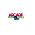## How to

When solving these problems, learning by doing is much better than learning by reading. I encourage to you read only as far in the solution as you need, then trying to solve the problem. If you get stuck, try reading a little further. And of course, let me know if you find a better solution!

## Wednesday, March 21, 2012

### Brainteaser: Turn a 6-sided dice into 5-sided dice

Problem:  Use a fair, six-sided die to play a game requiring a five-sided die.

Solution:  So, we're given one die as shown in the picture below.

There is nothing terribly special about this die; it's the standard type that is used in casino board games, etc, and we can assume that it's a fair die for this problem (not shaved, weighted to bias a number, etc.).

Unfortunately, when we roll it, it give us numbers from 1-6, not 1-5 as the problem requires.  What algorithm can we use that can allow us to generate random numbers?

Let's start by thinking about fairly obvious algorithms to transfer the range of numbers.

Some algorithms jump out immediately as fairly obvious.  One thing we could do is half the numbers and round up.  So, 1 and 2 could be 1.  3 and 4 could be 2.  5 and 6 could be 3.  This would give us numbers from 1-3 in a fair way.  Similarly, we can generate numbers from 1-2 by mapping numbers to range.

Unfortunately, there is no obvious way that we can map numbers and get to 5.

One other possibility is to roll multiple times.  For example, if we did two rolls, we would get a range between 2 and  12, though not an even range.  We could look at ways to divide the outputs of the dies (either the sum, or the sequence) and then slice it into fifths.  Perhaps we could even roll more than once until we found a distribution that could be sliced into fifths.  This could work, but the math very quickly gets tough and we end up with lots and lots of rolls.

Let's look again at the numbers that we can generate a range in so far:
2, 3 and 6.

Let's look at the 2, what does this mean:
Well, first, we generate this by assigning 1-3 to 1, and 4-6 to 2.
Seem familiar?  This is essentially a bit generator where we can generate a random bit each time where the range 1-3 produces the bit 0, and 4-6 the bit 1.

Now, how can we use a bit generator to generate a random number between 1-5?

Well, if you have a background in cryptography, this is easy.  If not, it's actually quite hard to see how this is helpful (though you can generate any range you want from this algorithm by making a big number and taking it % 5).  There is an easier way though.

So, let's go back to the die.  What else can we do when we roll it?  We looked at assigning ranges and adding, but what else can you do?  Do you need to use ever result of the die, or can you fairly ignore some?

How about ignoring a number?  Why not the number 6?

And there is the answer.  Roll the die like normal, and if you get a six, just re-roll.  There's your range, 1-5.

This is a good example of a class of brainteaser problems that appear to be complicated math, and in fact can be solved like that, but are actually quite simple.  When you get to seemingly really hard math, it's worth going back to your assumptions.  Your interviewer will generally comment that you're on the right path or not as well, so you can read the cluse.

/* Please let me know if you find alternative solutions or bugs.  Thanks, Noah */

1.This comment has been removed by the author.

2.The re-roll on 6 solution has the drawback of not having a deterministic runtime. It could (in theory) take an infinite number of rolls to get your result.

As far as I can tell, the only way to solve this problem is to roll enough times such that the sum of the maximum values are evenly divisible with the number of sides of the die you want to emulate, which in this case means five rolls.

3.I read your post and got it quite informative. I couldn't find any knowledge on this matter prior to. I would like to thanks for sharing this article here. If anyone looking to buy MTG Dice Counters online, Bluewizardgaming is the best choice.

4.